电工速算口诀：复杂电路中的电阻计算

信息来源：iiiccc.com  时间：2019-03-28  浏览次数：65

1. 概述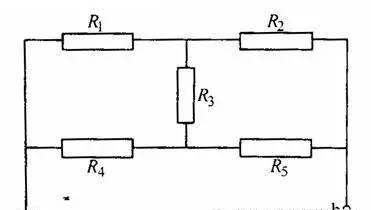2. 口诀说明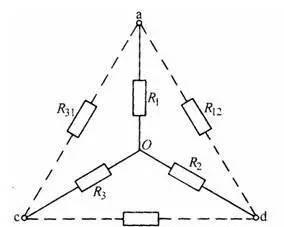（1）当由星形联结转换成三角形联结时，口诀为“星变角时求某边，两两积和除对面”。这里的“两两”是指星形联结时的每两个电阻，“两两积和”即为（R1R2+ R2R3+ R3R1）；“对面”是指与转换成三角形联结后的一个电阻相对的原星形联结的那个电阻，如图2中R12的“对面”应是R3。由此可得到由星形联结转换成三角形联结时的三个电阻计算公式为

R12＝（R1R2+ R2R3+ R3R1）/R3

R23＝（R1R2+ R2R3+ R3R1）/R1

R31＝（R1R2+ R2R3+ R3R1）/R2

（2）当由三角形联结转换成星形联结时，口诀为“角变星时求某枝，两臂之积除和三”。这里的“两臂”是指与转换成星形联结的一个电阻（后面称为“一枝”，例如R1）同一个顶点的三角形联结时的两个电阻（例如对应R1的两臂是R12和R31），“和三”即为三角形联结时三个电阻之和，即（R12+ R23+ R31）。由此可得到由三角形联结转换成星形联结时的三个电阻计算公式为

R1＝R12R31 /（R12+ R23+ R31）

R2＝R12R23 /（R12+ R23+ R31）

R3＝R23R31 /（R12+ R23+ R31）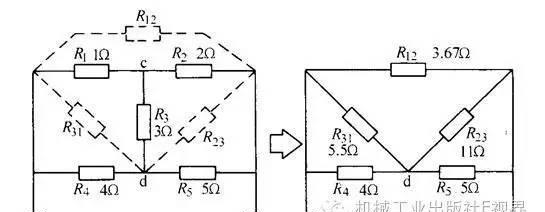a) 由星转换成角步骤1 b) 由星转换成角步骤2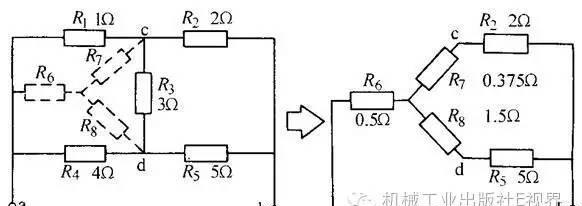c) 由角转换成星步骤1 d) 由角转换成星步骤2

（1）用由星形联结转换成三角形联结的方法

R1R2+ R2R3+ R3R1＝1×2+2×3+3×1=11

R12＝（R1R2+ R2R3+ R3R1）/R3=11/3=3.67Ω

R23＝（R1R2+ R2R3+ R3R1）/R1=11/1=11 Ω

R31＝（R1R2+ R2R3+ R3R1）/R2=11/2=5.5 Ω

Rab≈2.24Ω

（2）用由三角形联结转换成星形联结的方法

R1+ R3+ R4＝1+3+4＝8Ω

R6＝R1R4 /（R1+ R3+ R4）＝1×4/8＝0.5Ω

R7＝R1R3 /（R1+ R3+ R4）＝1×3/8＝0.375Ω

R8＝R3R4 /（R1+ R3+ R4）＝3×4/8＝1.5Ω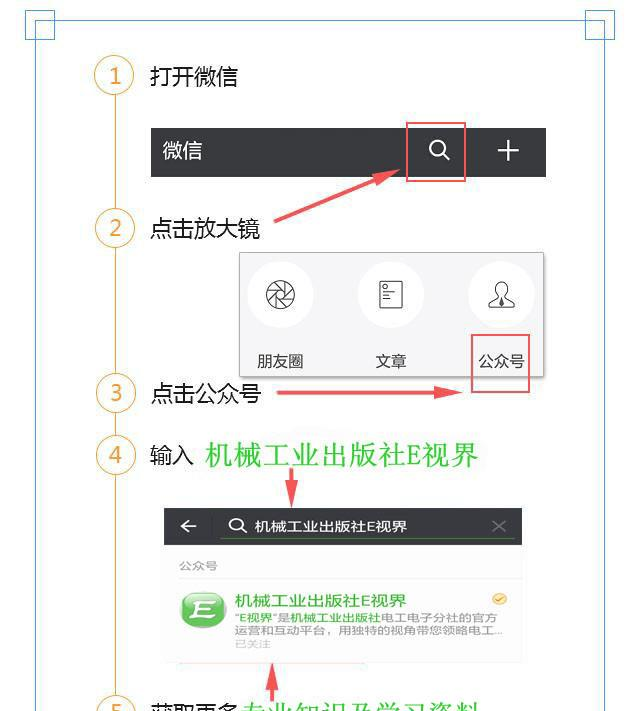——本信息真实性未经中国电路网证实，仅供您参考